### Python数据分析实战之北京二手房房价分析

``import pandas as pdimport numpy as npimport seaborn as snsimport matplotlib as mpl import matplotlib.pyplot as pltfrom IPython.display import displayplt.style.use("fivethirtyeight")sns.set_style({'font.sans-serif':['simhei','Arial']})%matplotlib inline  # 检查Python版本from sys import version_infoif version_info.major != 3:    raise Exception('请使用Python 3 来完成此项目')``

``# 导入链家二手房数据lianjia_df = pd.read_csv('lianjia.csv')display(lianjia_df.head(n=2))``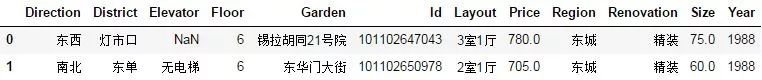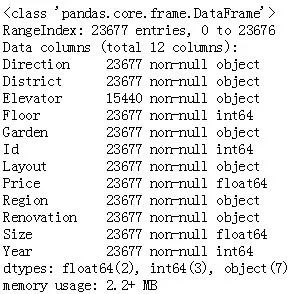``23677	Elevator``
``lianjia_df.describe()``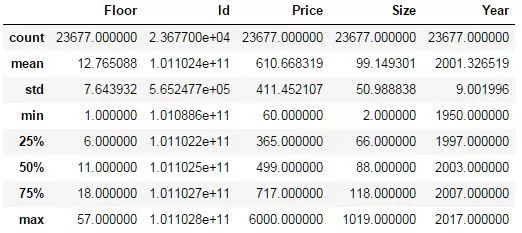``# 添加新特征房屋均价df = lianjia_df.copy()df['PerPrice'] = lianjia_df['Price']/lianjia_df['Size'] # 重新摆放列位置columns = ['Region', 'District', 'Garden', 'Layout', 'Floor', 'Year', 'Size', 'Elevator', 'Direction', 'Renovation', 'PerPrice', 'Price']df = pd.DataFrame(df, columns = columns) # 重新审视数据集display(df.head(n=2))``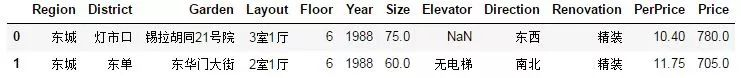Region特征分析

``# 对二手房区域分组对比二手房数量和每平米房价df_house_count = df.groupby('Region')['Price'].count().sort_values(ascending=False).to_frame().reset_index()df_house_mean = df.groupby('Region')['PerPrice'].mean().sort_values(ascending=False).to_frame().reset_index() f, [ax1,ax2,ax3] = plt.subplots(3,1,figsize=(20,15))sns.barplot(x='Region', y='PerPrice', palette="Blues_d", data=df_house_mean, ax=ax1)ax1.set_title('北京各大区二手房每平米单价对比',fontsize=15)ax1.set_xlabel('区域')ax1.set_ylabel('每平米单价') sns.barplot(x='Region', y='Price', palette="Greens_d", data=df_house_count, ax=ax2)ax2.set_title('北京各大区二手房数量对比',fontsize=15)ax2.set_xlabel('区域')ax2.set_ylabel('数量') sns.boxplot(x='Region', y='Price', data=df, ax=ax3)ax3.set_title('北京各大区二手房房屋总价',fontsize=15)ax3.set_xlabel('区域')ax3.set_ylabel('房屋总价') plt.show()``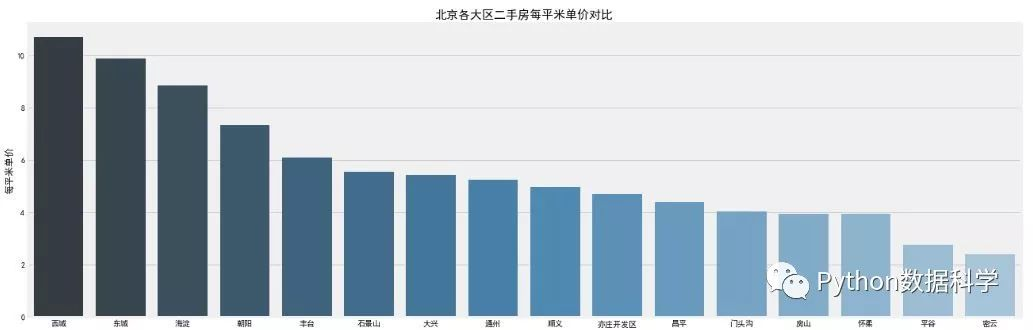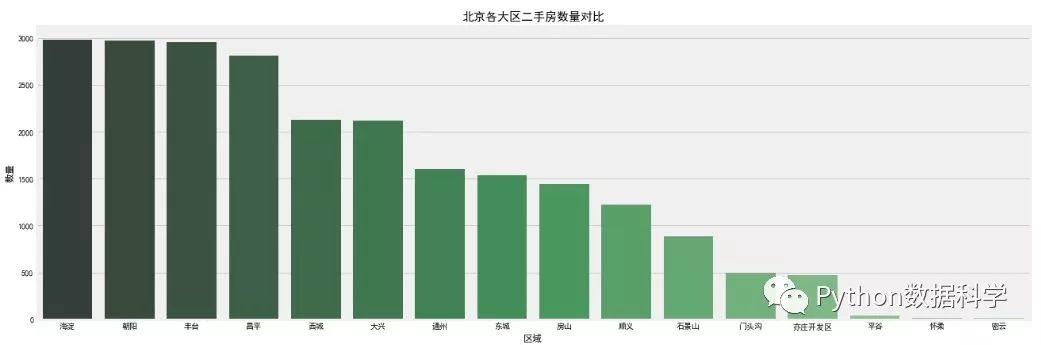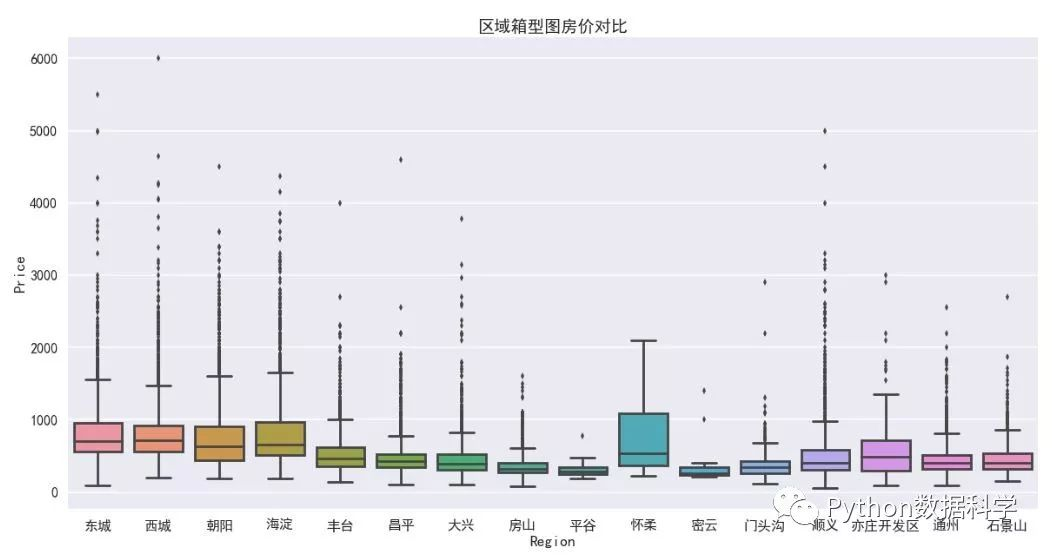• 二手房均价：西城区的房价最贵均价大约11万/平，因为西城在二环以里，且是热门学区房的聚集地。其次是东城大约10万/平，然后是海淀大约8.5万/平，其它均低于8万/平。

• 二手房房数量：从数量统计上来看，目前二手房市场上比较火热的区域。海淀区和朝阳区二手房数量最多，差不多都接近3000套，毕竟大区，需求量也大。然后是丰台区，近几年正在改造建设，有赶超之势。

• 二手房总价：通过箱型图看到，各大区域房屋总价中位数都都在1000万以下，且房屋总价离散值较高，西城最高达到了6000万，说明房屋价格特征不是理想的正太分布。

Size特征分析

``f, [ax1,ax2] = plt.subplots(1, 2, figsize=(15, 5))# 建房时间的分布情况sns.distplot(df['Size'], bins=20, ax=ax1, color='r')sns.kdeplot(df['Size'], shade=True, ax=ax1)# 建房时间和出售价格的关系sns.regplot(x='Size', y='Price', data=df, ax=ax2)plt.show()``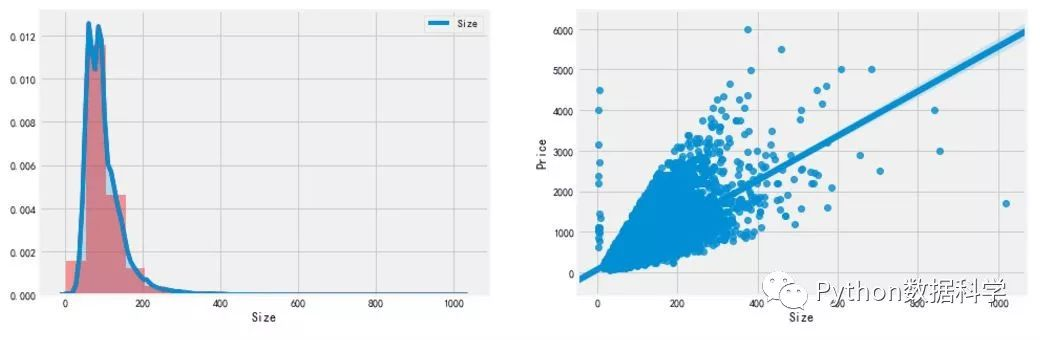• Size 分布：

通过 distplot  和 kdeplot 绘制柱状图观察 Size 特征的分布情况，属于长尾类型的分布，这说明了有很多面积很大且超出正常范围的二手房。

• Size 与 Price 的关系：

通过 regplot 绘制了 Size 和 Price 之间的散点图，发现 Size 特征基本与Price呈现线性关系，符合基本常识，面积越大，价格越高。但是有两组明显的异常点：1. 面积不到10平米，但是价格超出10000万；2. 一个点面积超过了1000平米，价格很低，需要查看是什么情况。

``df.loc[df['Size']&lt; 10]``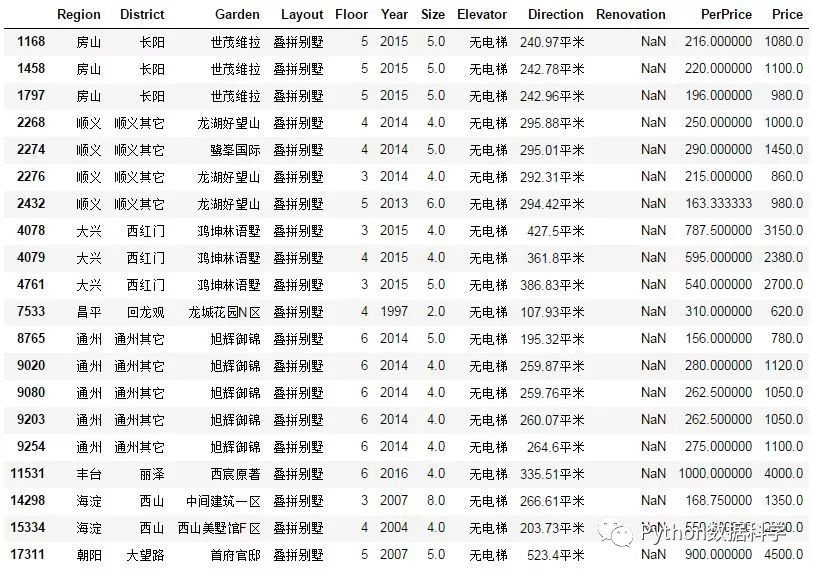``````df.loc[df['Size']&gt;1000]
``````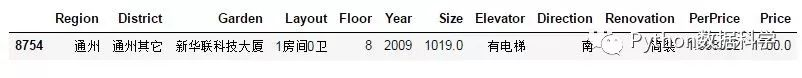``df = df[(df['Layout']!='叠拼别墅')&amp;(df['Size']&lt;1000)]``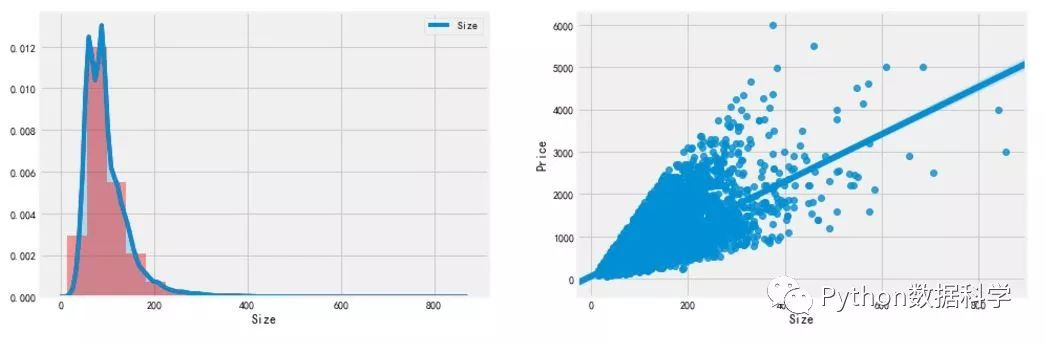Layout特征分析

``f, ax1= plt.subplots(figsize=(20,20))sns.countplot(y='Layout', data=df, ax=ax1)ax1.set_title('房屋户型',fontsize=15)ax1.set_xlabel('数量')ax1.set_ylabel('户型')plt.show()``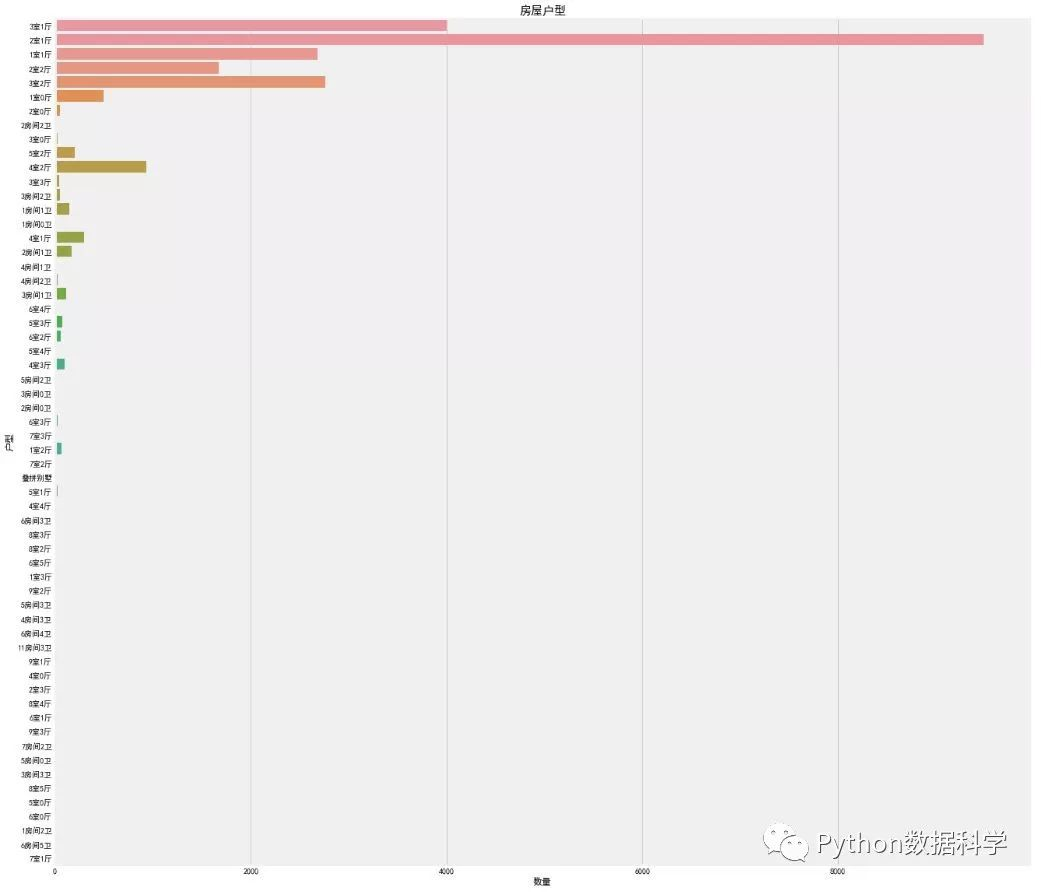Renovation 特征分析

``# 去掉错误数据“南北”，因为爬虫过程中一些信息位置为空，导致“Direction”的特征出现在这里，需要清除或替换df['Renovation'] = df.loc[(df['Renovation'] != '南北'), 'Renovation'] # 画幅设置f, [ax1,ax2,ax3] = plt.subplots(1, 3, figsize=(20, 5))sns.countplot(df['Renovation'], ax=ax1)sns.barplot(x='Renovation', y='Price', data=df, ax=ax2)sns.boxplot(x='Renovation', y='Price', data=df, ax=ax3)plt.show()``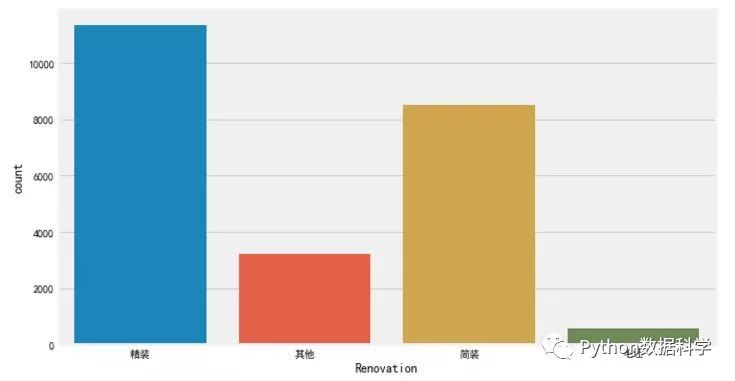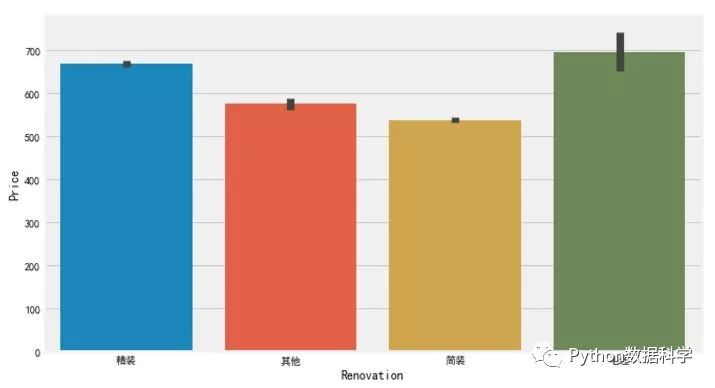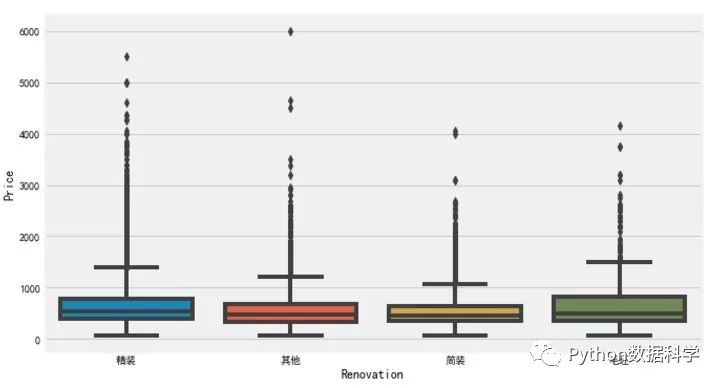Elevator 特征分析

``misn = len(df.loc[(df['Elevator'].isnull()), 'Elevator'])print('Elevator缺失值数量为：'+ str(misn))``

Elevator 缺失值数量为：8237

``# 由于存在个别类型错误，如简装和精装，特征值错位，故需要移除df['Elevator'] = df.loc[(df['Elevator'] == '有电梯')|(df['Elevator'] == '无电梯'), 'Elevator'] # 填补Elevator缺失值df.loc[(df['Floor']&gt;6)&amp;(df['Elevator'].isnull()), 'Elevator'] = '有电梯'df.loc[(df['Floor']&lt;=6)&amp;(df['Elevator'].isnull()), 'Elevator'] = '无电梯' f, [ax1,ax2] = plt.subplots(1, 2, figsize=(20, 10))sns.countplot(df['Elevator'], ax=ax1)ax1.set_title('有无电梯数量对比',fontsize=15)ax1.set_xlabel('是否有电梯')ax1.set_ylabel('数量')sns.barplot(x='Elevator', y='Price', data=df, ax=ax2)ax2.set_title('有无电梯房价对比',fontsize=15)ax2.set_xlabel('是否有电梯')ax2.set_ylabel('总价')plt.show()``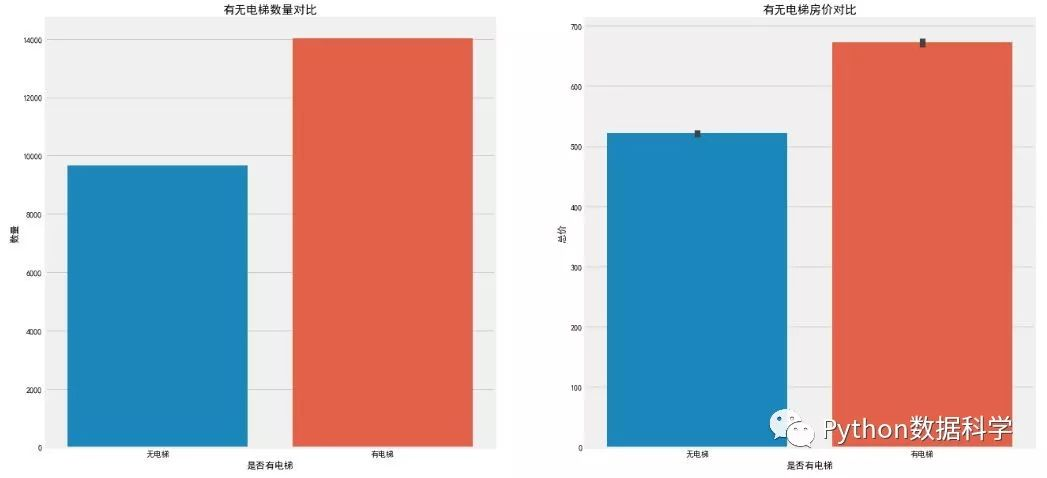Year 特征分析

``grid = sns.FacetGrid(df, row='Elevator', col='Renovation', palette='seismic',size=4)grid.map(plt.scatter, 'Year', 'Price')grid.add_legend()``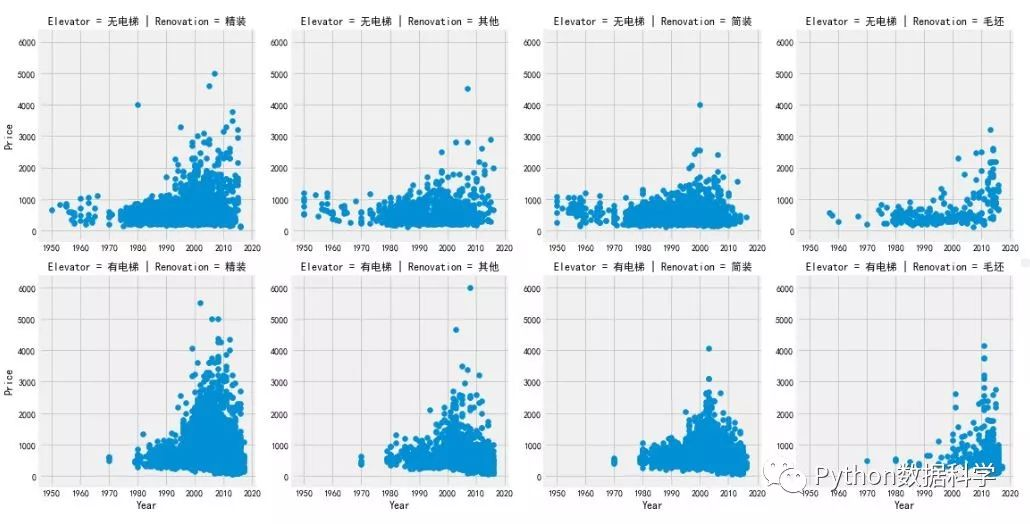• 整个二手房房价趋势是随着时间增长而增长的；

• 2000年以后建造的二手房房价相较于2000年以前有很明显的价格上涨；

• 1980年之前几乎不存在有电梯二手房数据，说明1980年之前还没有大面积安装电梯；

• 1980年之前无电梯二手房中，简装二手房占绝大多数，精装反而很少；

Floor 特征分析

``f, ax1= plt.subplots(figsize=(20,5))sns.countplot(x='Floor', data=df, ax=ax1)ax1.set_title('房屋户型',fontsize=15)ax1.set_xlabel('数量')ax1.set_ylabel('户型')plt.show()``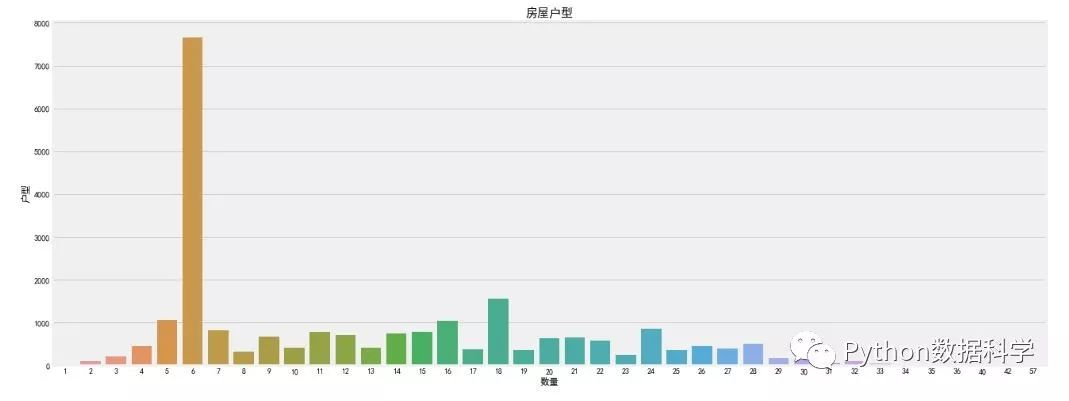• 解决爬虫获取的数据源准确度问题；

• 需要爬取或者寻找更多好的售房特征；

• 需要做更多地特征工程工作，比如数据清洗，特征选择和筛选；

• 使用统计模型建立回归模型进行价格预测；Python能用来做什么？以下是Python的三大主要用途

Python爬虫实战（批量采集Stock数据，并保存到Excel中）